Ex 11.1

Chapter 11 Class 8 Mensuration
Serial order wise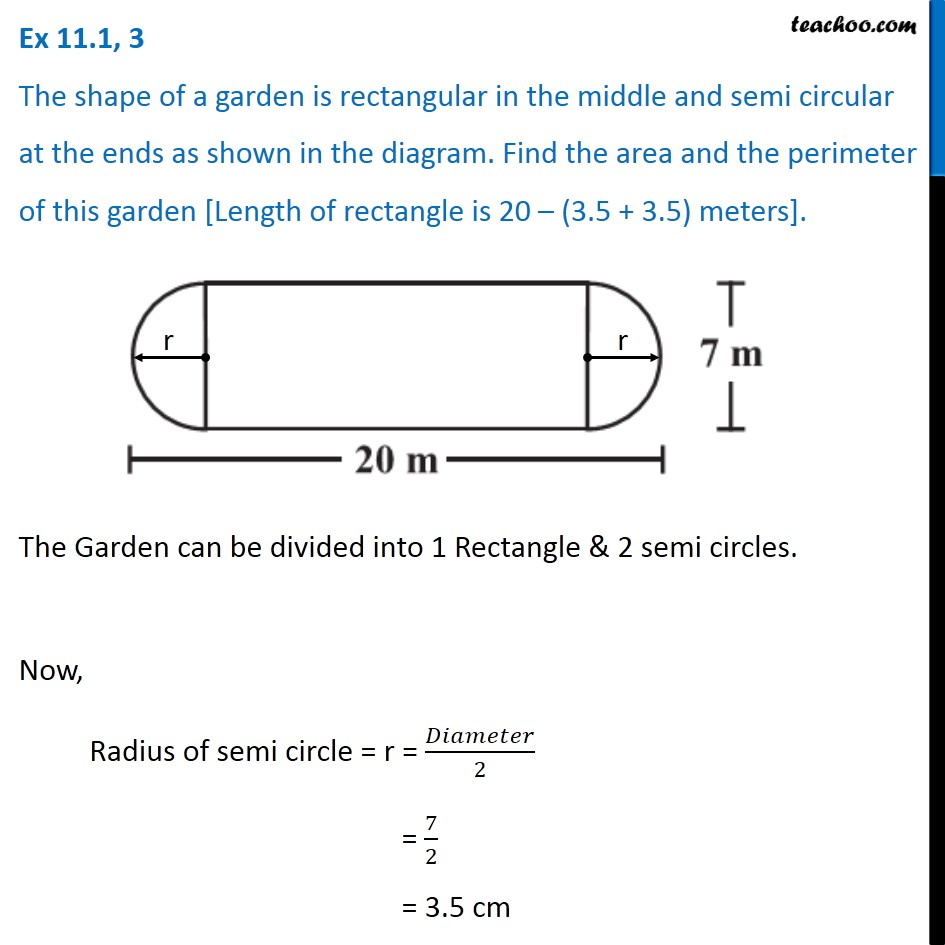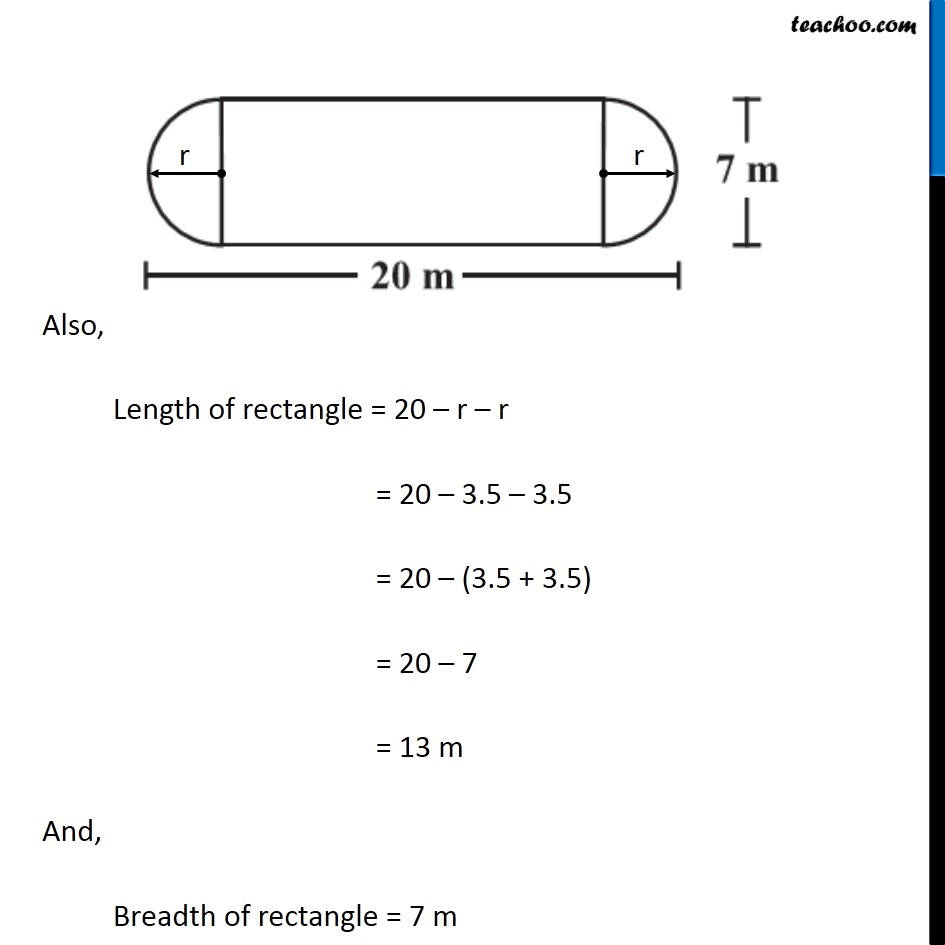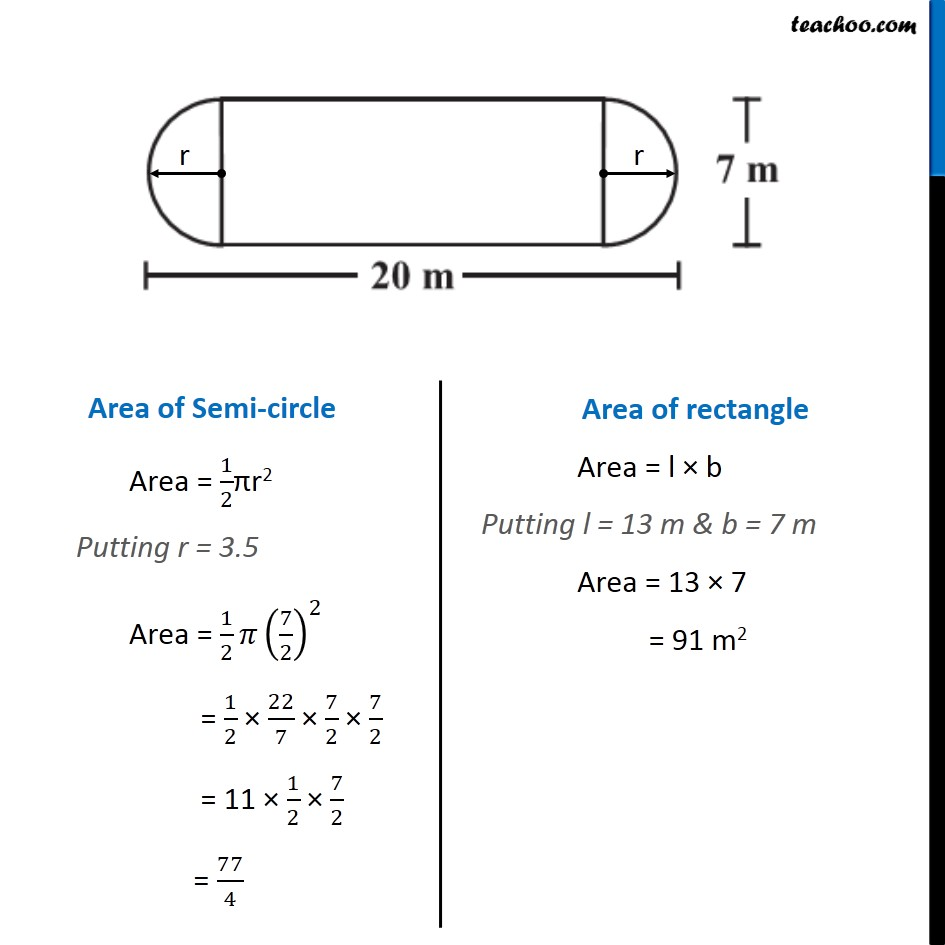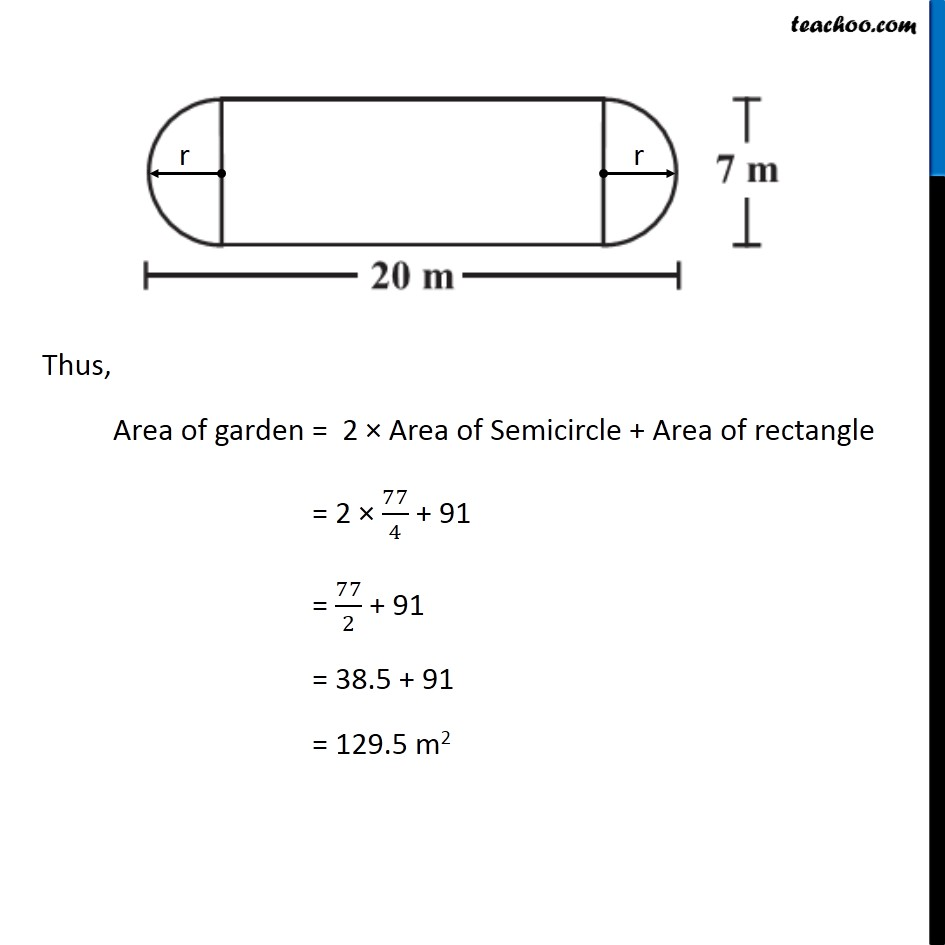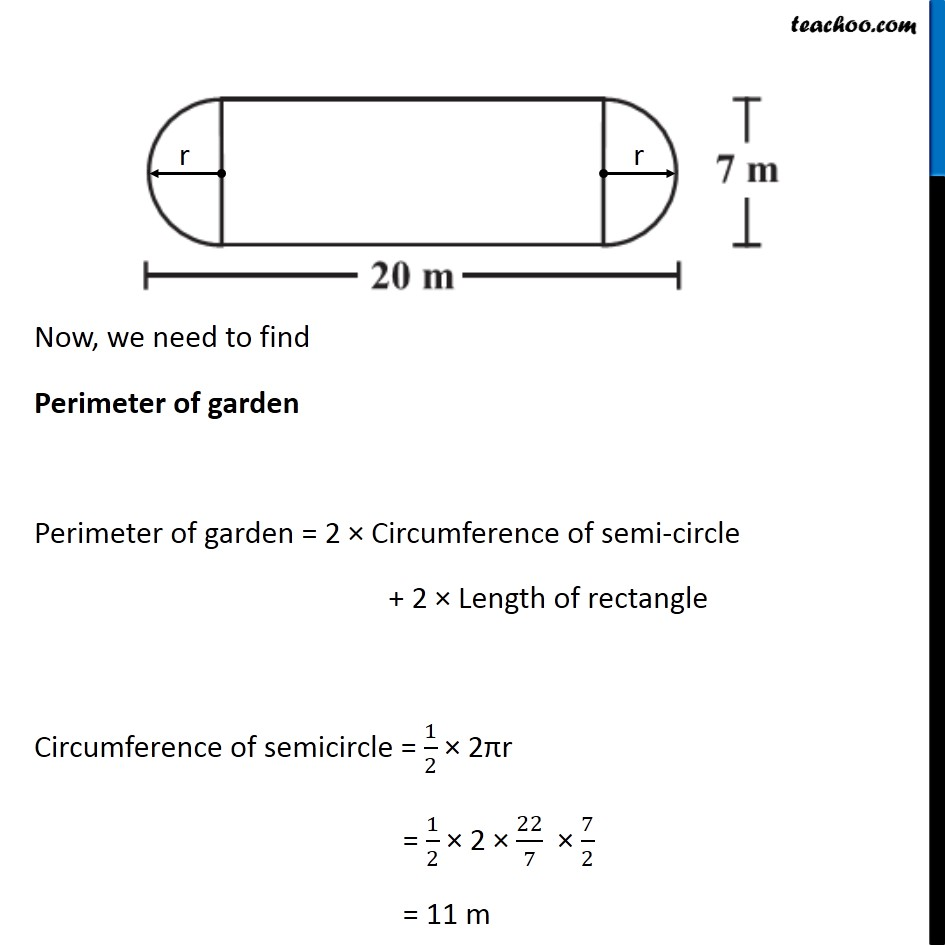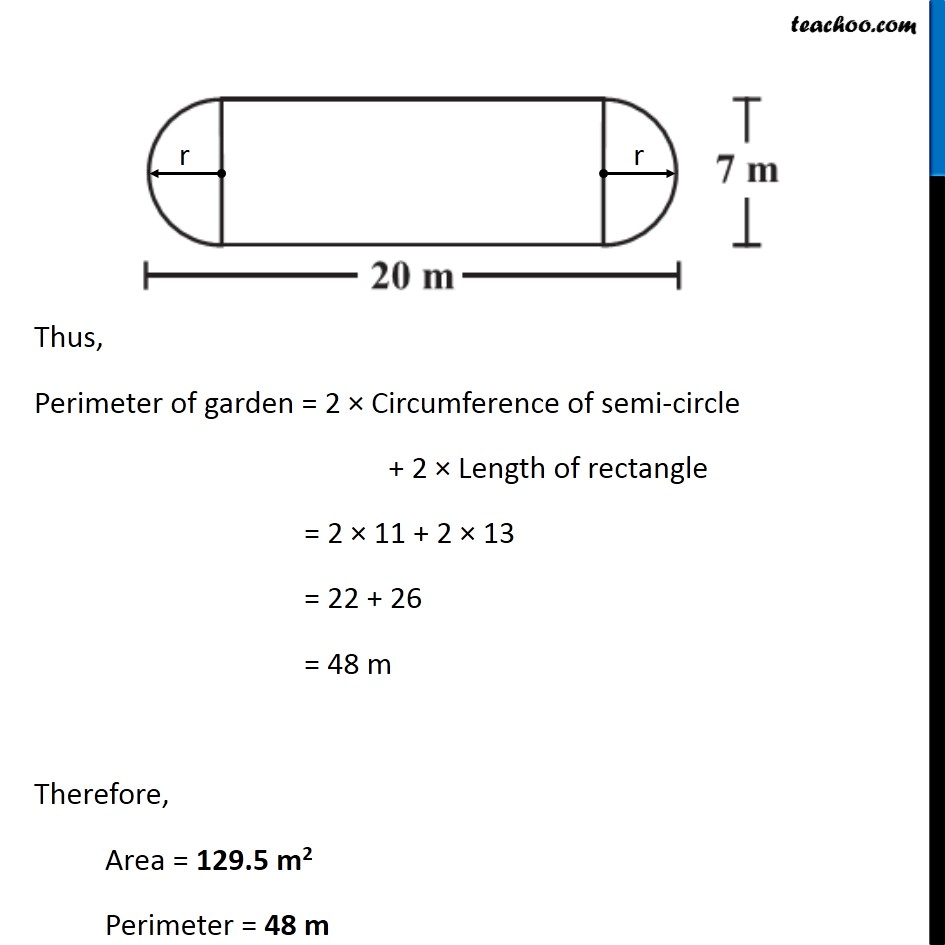### Transcript

Ex 11.1, 3 The shape of a garden is rectangular in the middle and semi circular at the ends as shown in the diagram. Find the area and the perimeter of this garden [Length of rectangle is 20 – (3.5 + 3.5) meters]. The Garden can be divided into 1 Rectangle & 2 semi circles. Now, Radius of semi circle = r = 𝐷𝑖𝑎𝑚𝑒𝑡𝑒𝑟/2 = 7/2 = 3.5 cm Also, Length of rectangle = 20 – r – r = 20 – 3.5 – 3.5 = 20 – (3.5 + 3.5) = 20 – 7 = 13 m And, Breadth of rectangle = 7 m Area of Semi-circle Area = 1/2πr2 Putting r = 3.5 Area = 1/2 𝜋(7/2)^2 = 1/2 × 22/7 × 7/2 × 7/2 = 11 × 1/2 × 7/2 = 77/4 Area of rectangle Area = l × b Putting l = 13 m & b = 7 m Area = 13 × 7 = 91 m2 Thus, Area of garden = 2 × Area of Semicircle + Area of rectangle = 2 × 77/4 + 91 = 77/2 + 91 = 38.5 + 91 = 129.5 m2 Now, we need to find Perimeter of garden Perimeter of garden = 2 × Circumference of semi-circle + 2 × Length of rectangle Circumference of semicircle = 1/2 × 2πr = 1/2 × 2 × 22/7 × 7/2 = 11 m Now, we need to find Perimeter of garden Perimeter of garden = 2 × Circumference of semi-circle + 2 × Length of rectangle Circumference of semicircle = 1/2 × 2πr = 1/2 × 2 × 22/7 × 7/2 = 11 m